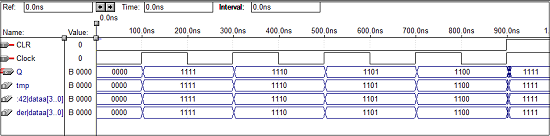# VHDL Programming for Sequential Circuits

This chapter explains how to do VHDL programming for Sequential Circuits.

## VHDL Code for an SR Latch

library ieee;
use ieee.std_logic_1164.all;

entity srl is
port(r,s:in bit; q,qbar:buffer bit);
end srl;

architecture virat of srl is
signal s1,r1:bit;
begin
q<= s nand qbar;
qbar<= r nand q;
end virat;


### Waveforms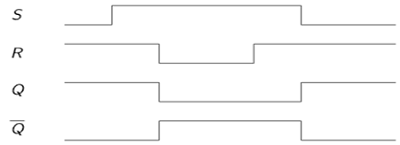## VHDL Code for a D Latch

library ieee;
use ieee.std_logic_1164.all;

entity Dl is
port(d:in bit; q,qbar:buffer bit);
end Dl;

architecture virat of Dl is
signal s1,r1:bit;
begin
q<= d nand qbar;
qbar<= d nand q;
end virat;


### Waveforms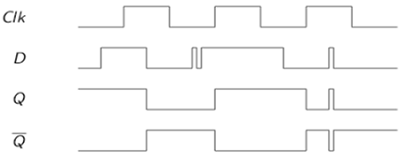## VHDL Code for an SR Flip Flop

library ieee;
use ieee.std_logic_1164.all;

entity srflip is
port(r,s,clk:in bit; q,qbar:buffer bit);
end srflip;

architecture virat of srflip is
signal s1,r1:bit;
begin
s1<=s nand clk;
r1<=r nand clk;
q<= s1 nand qbar;
qbar<= r1 nand q;
end virat;


### Waveforms## VHDL code for a JK Flip Flop

library IEEE;
use IEEE.STD_LOGIC_1164.all;

entity jk is
port(
j : in STD_LOGIC;
k : in STD_LOGIC;
clk : in STD_LOGIC;
reset : in STD_LOGIC;
q : out STD_LOGIC;
qb : out STD_LOGIC
);
end jk;

architecture virat of jk is
begin
jkff : process (j,k,clk,reset) is
variable m : std_logic := '0';

begin
if (reset = '1') then
m : = '0';
elsif (rising_edge (clk)) then
if (j/ = k) then
m : = j;
elsif (j = '1' and k = '1') then
m : = not m;
end if;
end if;

q <= m;
qb <= not m;
end process jkff;
end virat;


### Waveforms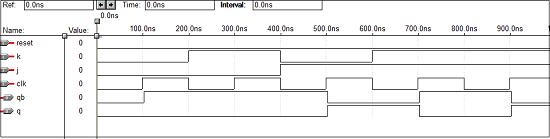## VHDL Code for a D Flip Flop

Library ieee;
use ieee.std_logic_1164.all;

entity dflip is
port(d,clk:in bit; q,qbar:buffer bit);
end dflip;

architecture virat of dflip is
signal d1,d2:bit;
begin
d1<=d nand clk;
d2<=(not d) nand clk;
q<= d1 nand qbar;
qbar<= d2 nand q;
end virat;


### Waveforms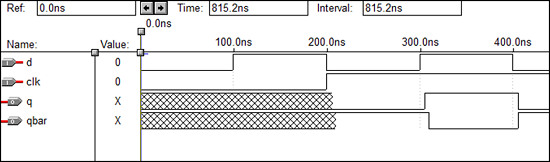## VHDL Code for a T Flip Flop

library IEEE;
use IEEE.STD_LOGIC_1164.all;

entity Toggle_flip_flop is
port(
t : in STD_LOGIC;
clk : in STD_LOGIC;
reset : in STD_LOGIC;
dout : out STD_LOGIC
);
end Toggle_flip_flop;

architecture virat of Toggle_flip_flop is
begin
tff : process (t,clk,reset) is
variable m : std_logic : = '0';

begin
if (reset = '1') then
m : = '0';
elsif (rising_edge (clk)) then
if (t = '1') then
m : = not m;
end if;
end if;
dout < = m;
end process tff;
end virat;


### Waveforms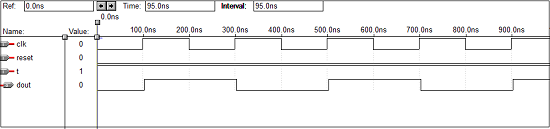## VHDL Code for a 4 - bit Up Counter

library IEEE;
use ieee.std_logic_1164.all;
use ieee.std_logic_unsigned.all;

entity counter is
port(Clock, CLR : in std_logic;
Q : out std_logic_vector(3 downto 0)
);
end counter;

architecture virat of counter is
signal tmp: std_logic_vector(3 downto 0);
begin
process (Clock, CLR)

begin
if (CLR = '1') then
tmp < = "0000";
elsif (Clock'event and Clock = '1') then
mp <= tmp + 1;
end if;
end process;
Q <= tmp;
end virat;


### Waveforms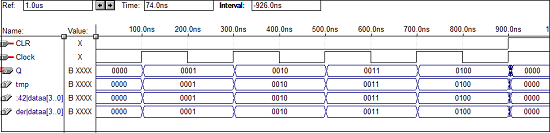## VHDL Code for a 4-bit Down Counter

library ieee;
use ieee.std_logic_1164.all;
use ieee.std_logic_unsigned.all;

entity dcounter is
port(Clock, CLR : in std_logic;
Q : out std_logic_vector(3 downto 0));
end dcounter;

architecture virat of dcounter is
signal tmp: std_logic_vector(3 downto 0);

begin
process (Clock, CLR)
begin
if (CLR = '1') then
tmp <= "1111";
elsif (Clock'event and Clock = '1') then
tmp <= tmp - 1;
end if;
end process;
Q <= tmp;
end virat;


### Waveforms